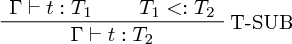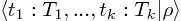## Row Polymorphism Isn't Subtyping

I’ve been working on Roy’s new type system which uses a concept called row variables. I’ve also been thinking about TypeScript’s unsound variance issues with its implementation of structural subtyping. That lead to an interesting discussion on Twitter about row polymorphism compared to structural subtyping.

Both are trying to type code like the following:

``````let f x = x.a + x.b
f {a: 1, b: 2, c: 100}``````

Also known as static duck typing.

Structural subtyping of course uses subtyping to achieve the above. Subtyping involves the rule of subsumption:In English: if we have a term `t` in our environment of type `T1` and `T1` is a subtype of `T2`, then that term also has type `T2`.

In structural subtyping, a wider record type is subsumed by a narrower one. Due to contravariance of function inputs, we can supply any subsumed record to `f`:

``f :: {a: Number, b: Number} -> Number``

Row polymorphism instead achieves the original code is by allowing records to have a special polymorphic variable:The rho (ρ) represents a variable which can be instantiated to extra fields. That means the type of `f` is:

``f :: {a: Number, b: Number | ρ} -> Number``

And when we apply `f`, `ρ` is instantiated to contain the extra field, `c: Number`.

So now to answer the question, is row polymorphism a form of subtyping? No, because of the instantiation of the row variable, we can’t derive the rule of subsumption. It would need a separate instantiation step defined and that means there is no subtyping relationship.

Here’s a simple example of where the row instantiation can cause differences:

``````let f x = x with {sum: x.a + x.b}
let answer = f {a: 1, b: 2, c: 100}``````

With structural subtyping, the type is upcast to what `f` needs and we get back:

``answer :: {a: Number, b: Number, sum: Number}``

But with row polymorphism, the row variable `ρ` gets instantiated to contain the `c` field:

``answer :: {a: Number, b: Number, c: Number, sum: Number}``

Great. Row polymorphism isn’t subtyping. Who cares? Well, subtyping and type inference just don’t mix. Doing type inference with row polymorphic records is much easier. There’s still undecidability problems but if you’re writing a language with type inference and want records, you should take a look at row variables.